# On a \$1000 loan at 20% interest, why is my interest not \$200 for one year?

Q: On a \$1000 loan at 20% interest, why is my interest not \$200 for one year?

A: This is a common question that we often get and some information is missing to answer the question so we’ll analyse this, taking into account various scenarios and how to manage this in Margill Loan Manager.

There is a misunderstanding as to the concept of “amortization”.

Here is how we get to \$200 in interest on a loan. It must have ONE (1) lump sum payment at the end (one year later) of 1200 to get a balance of 0.00. So there is no amortization in this loan: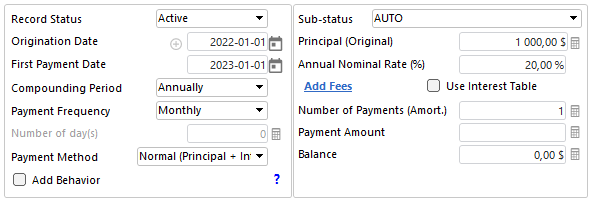Compute to get the Results table: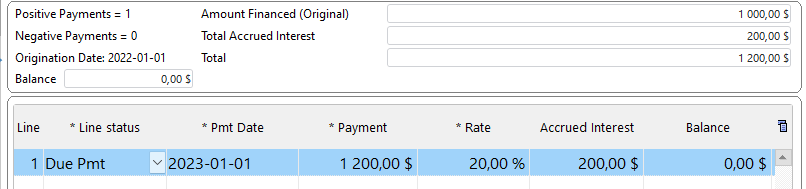Let’s look at this with bi-weekly \$0.00 payments just to see the interest accrued (so 26 payments and the last payment on Jan. 1, 2023 to give exactly one year). This is Compound interest (not Simple interest), so the interest keeps on increasing: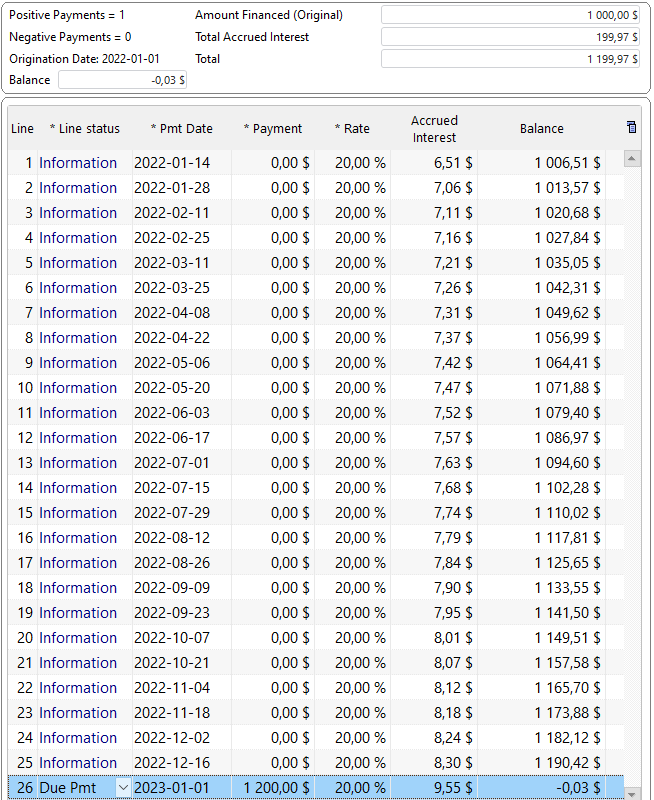So you get exactly 200 (+ or – a few cents due to rounding) as the interest amount.
However, when you add true payments that pay interest and principal (every 2 weeks, so 26 for a full year approximately), you are not lending 1000 for 1 year since principal gets paid back every 2 weeks, thus reducing the interest accrued.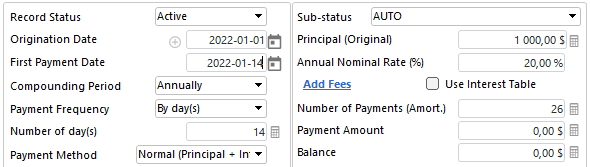“Compute” to get a real amortization schedule at 20% annual (APR). Notice my balance goes down so the fortnightly interest (every 2 weeks) goes down and so does the interest per period. So for an amortized loan, the interest is very far from 200 total, only about half (96.96) because of the amortization effect.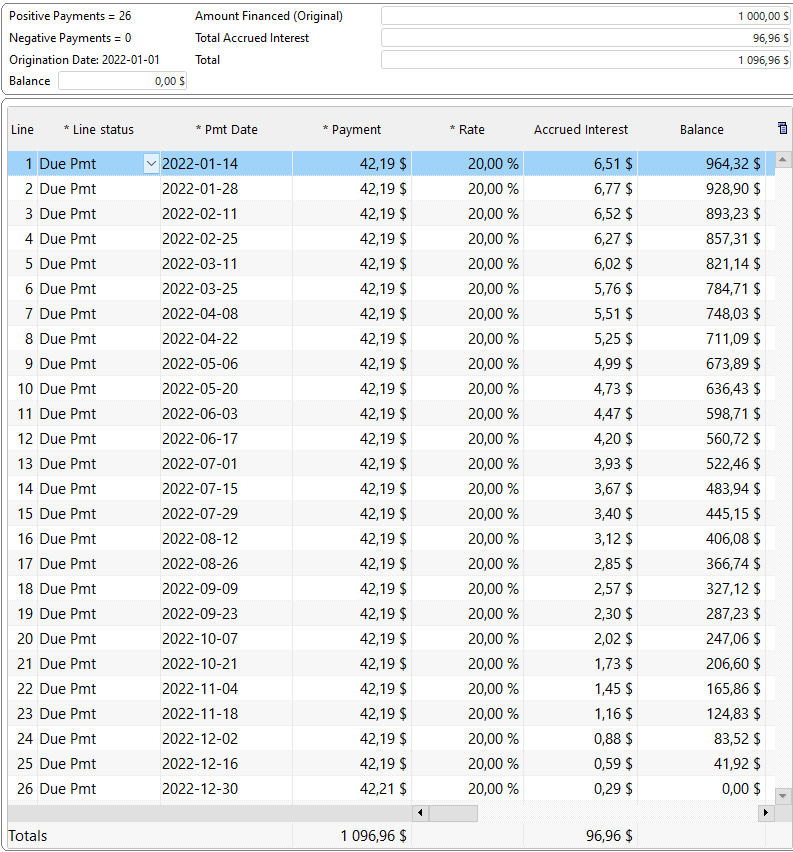There are two ways to get the desired \$200 in accrued interest for 1 year when there are true principal and interest (P&I) payments:
Method 1): Calculate the REAL interest rate
• Desired Interest per payment: 200 / 26 = 7.69
• Principal per payment: 1000 / 26 = 38.46
• So 26 payments of 46.15 each (26 x 46.15 = 1199.90)
Leave the Annual Nominal Rate blank and enter the Payment of 46.15. Margill will compute the rate.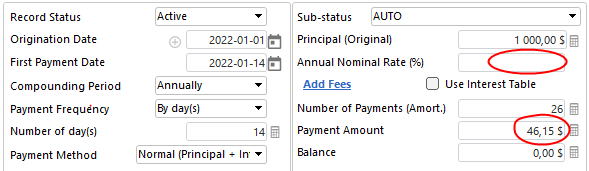“Compute” and notice the real interest rate (APR) is now 43.97% (APR). We are at 199.90 in interest (almost 200).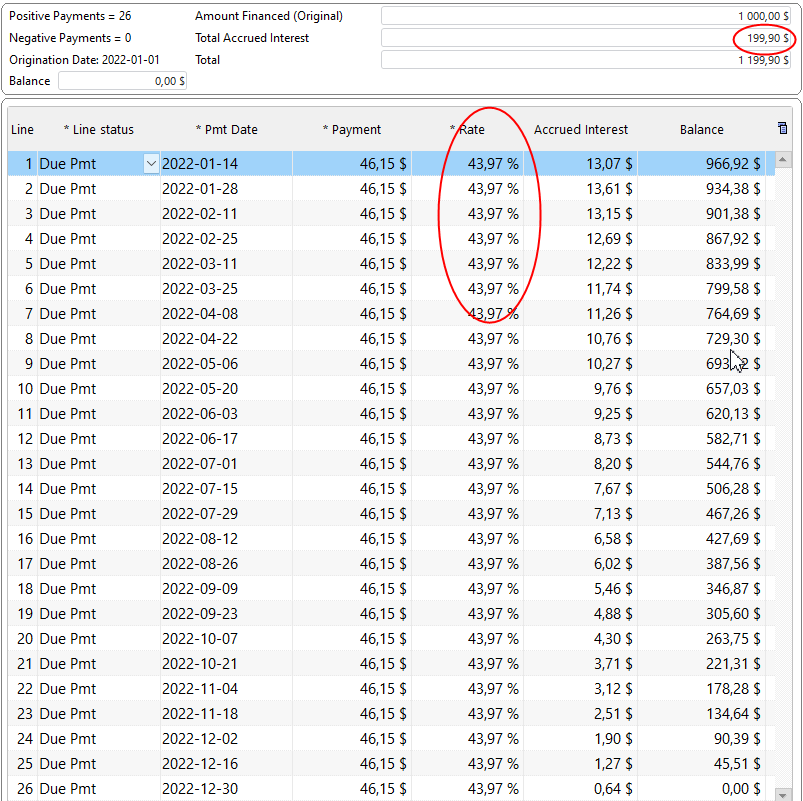2) Use Fees, not true interest
Other option is to use Column fees (that are not computed on a daily basis but entered once and no matter what, you will have 200 in “finance costs”, not real interest). Click on Add Fees (I called them Admin fees – you can rename them to anything you want) and add 7.69 (200 / 26) in “interest” (Admin Fees here) per payment.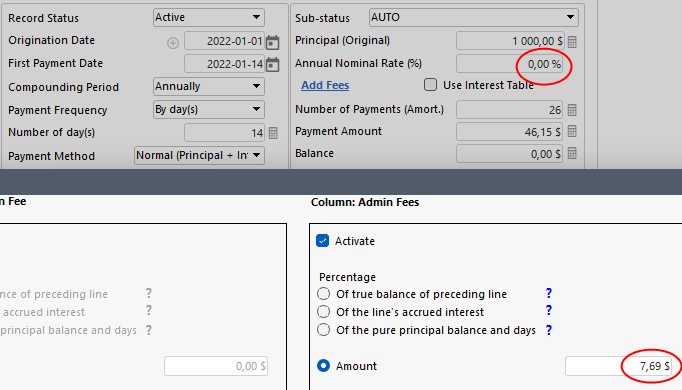Here are the results. I added a few cents in Admin Fees at the end and increased my payment to get exactly 200 as my finance cost. Notice my interest rate is 0% since I am now using Column fees, not real interest.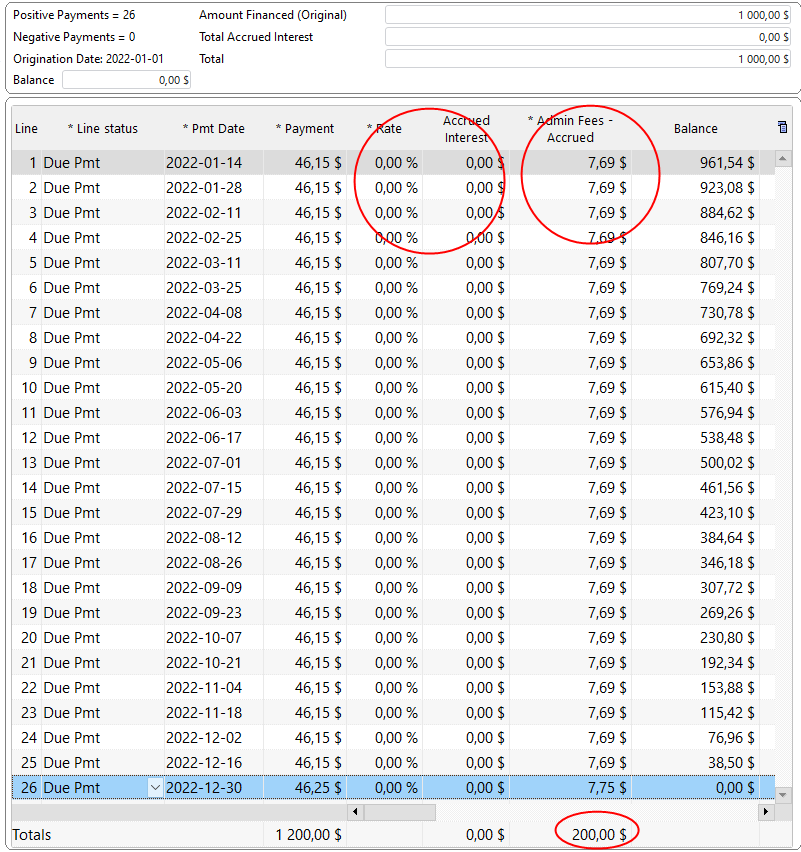I also invite you to consult our White Paper on interest. It explains basics and more advanced issues with interest: https://www.margill.com/en/interest-calculation-white-paper/